# phased.CustomAntennaElement

Custom antenna element

## Description

The `phased.CustomAntennaElement` System object™ models an antenna element with a custom spatial response pattern. The response pattern can be defined for polarized or non-polarized fields.

To create a custom antenna element:

1. Create the `phased.CustomAntennaElement` object and set its properties.

2. Call the object with arguments, as if it were a function.

## Creation

### Syntax

``antenna = phased.CustomAntennaElement``
``antenna = phased.CustomAntennaElement(Name,Value)``

### Description

````antenna = phased.CustomAntennaElement` creates a System object, `antenna`, with default property values. The default response pattern is spatially isotropic.```

example

````antenna = phased.CustomAntennaElement(Name,Value)` creates a custom antenna object, `antenna`, with each specified property Name set to the specified Value. You can specify additional name-value pair arguments in any order as (`Name1`,`Value1`,...,`NameN`,`ValueN`). For example, the output response of the object depends on whether polarization is set or not. To create a nonpolarized response pattern, set the `SpecifyPolarizationPattern` property to `false` (default). Then, use the `MagnitudePattern` and `PhasePattern` properties to define the response pattern.To create a polarized response pattern, set the `SpecifyPolarizationPattern` property to `true`. Then, use any or all of the `HorizontalMagnitudePattern`, `HorizontalPhasePattern`, `VerticalMagnitudePattern`, and `VerticalPhasePattern` properties to define the response pattern. ```

## Properties

expand all

Unless otherwise indicated, properties are nontunable, which means you cannot change their values after calling the object. Objects lock when you call them, and the `release` function unlocks them.

If a property is tunable, you can change its value at any time.

Frequencies at which the frequency response and antenna patterns are to be returned, specified as a 1-by-L row vector. The elements of the vector must be in increasing order. The antenna element has no response outside the frequency range specified by the minimum and maximum elements of the frequency vector. Units are in Hz.

Example: `[200:50:300]*1e6`

Data Types: `double`

Frequency responses at the frequencies defined in `FrequencyVector` property, specified as a 1-by-L row vector. L equals the length of the vector specified in the `FrequencyVector` property. Units are in dB.

Example: `[0 6 0]`

Data Types: `double`

Coordinate system of custom antenna pattern, specified `'az-el'` or `'phi-theta'`. When you specify `'az-el'`, use the `AzimuthAngles` and `ElevationAngles` properties to specify the pattern coordinates system. When you specify `'phi-theta'`, use the `PhiAngles` and `ThetaAngles` properties to specify the pattern coordinates system.

Data Types: `char`

Specify the azimuth angles as a length-P vector. These angles are the azimuth angles where the custom radiation pattern is specified. P must be greater than 2. The azimuth angles must lie between –180° and 180° and be in strictly increasing order. Units are in degrees.

Example: `[30 40 50]`

#### Dependencies

To enable this property, set the `PatternCoordinateSystem` property to `'az-el'`.

Data Types: `double`

Specify the elevation angles as a length-Q vector. These angles are the elevation angles where the custom radiation pattern is specified. Q must be greater than 2. The elevation angles must lie between –90° and 90° and be in strictly increasing order. Units are in degrees.

Example: `[-30 0 +30]`

#### Dependencies

To enable this property, set the `PatternCoordinateSystem` property to `'az-el'`.

Data Types: `double`

Phi angles in phi-theta coordinate system, specified as a real-valued P-length vector. These angles are the phi angles where the custom pattern is specified. P must be greater than 2.

Example: `[90:180]`

#### Dependencies

To enable this property, set the `PatternCoordinateSystem` property to `'phi-theta'`.

Data Types: `double`

Theta angles in phi-theta coordinate system, specified as a real-valued Q-length vector. These angles are the theta angles where the custom pattern is specified. Q must be greater than 2.

Example: `[40:80]`

#### Dependencies

To enable this property, set the `PatternCoordinateSystem` property to `'phi-theta'`.

Data Types: `double`

Polarized array response, specified as `false` or `true`.

• When the `SpecifyPolarizationPattern` property is set to `false`, the antenna element transmits or receives non-polarized radiation. In this case, use the `MagnitudePattern` property to set the antenna response pattern.

• When the `SpecifyPolarizationPattern` property is set to `true`, the antenna element transmits or receives polarized radiation. In this case, use the `HorizontalMagnitudePattern` and `HorizontalPhasePattern` properties to set the horizontal polarization response pattern and the `VerticalMagnitudePattern` and `VerticalPhasePattern` properties to set the vertical polarization response pattern.

Data Types: `logical`

The magnitude of the combined polarization antenna radiation, pattern specified as a Q-by-P matrix or a Q-by-P-by-L array. This property is used only when the `SpecifyPolarizationPattern` property is set to `false`. Magnitude units are in dB.

• If the value of this property is a Q-by-P matrix, the same pattern is applied to all frequencies specified in the `FrequencyVector` property.

• If the value is a Q-by-P-by-L array, each Q-by-P page of the array specifies a pattern for the corresponding frequency specified in the `FrequencyVector` property.

If the pattern contains a `NaN` at any azimuth and elevation direction, it is converted to `-Inf`, indicating zero response in that direction. The custom antenna object uses interpolation to estimate the response of the antenna at a given direction. To avoid interpolation errors, the custom response pattern must contain azimuth angles in the range `[–180,180]` degrees. Set the range of elevation angles to `[–90,90]` degrees.

Data Types: `double`

The phase of the combined polarization antenna radiation pattern, specified as a Q-by-P matrix or a Q-by-P-by-L array. This property is used only when the `SpecifyPolarizationPattern` property is set to `false`. Units are in degrees.

• If the value of this property is a Q-by-P matrix, the same pattern is applied to all frequencies specified in the `FrequencyVector` property.

• If the value is a Q-by-P-by-L array, each Q-by-P page of the array specifies a pattern for the corresponding frequency specified in the `FrequencyVector` property.

The custom antenna object uses interpolation to estimate the response of the antenna at a given direction. To avoid interpolation errors, the custom response pattern must contain azimuth angles in the range [–180°,180°]. Set the range of elevation angles to [–90°,90°].

Data Types: `double`

The magnitude of the horizontal polarization component of the antenna radiation pattern, specified as a real-valued Q-by-P matrix or real-valued a Q-by-P-by-L array. Magnitude units are in dB.

• If the value of this property is a Q-by-P matrix, the same pattern is applied to all frequencies specified in the `FrequencyVector` property.

• If the value is a Q-by-P-by-L array, each Q-by-P page of the array specifies a pattern for the corresponding frequency specified in the `FrequencyVector` property.

If the magnitude pattern contains a `NaN` at any azimuth and elevation direction, it is converted to `-Inf`, indicating zero response in that direction. The custom antenna object uses interpolation to estimate the response of the antenna at a given direction. To avoid interpolation errors, the custom response pattern must contain azimuth angles in the range `[–180,180]°` and elevation angles in the range `[–90,90]°`.

#### Dependencies

To enable this property, set the `SpecifyPolarizationPattern` property to `true`.

Data Types: `double`

The phase of the horizontal polarization component of the antenna radiation pattern, specified as a real-valued Q-by-P matrix or a real-valued Q-by-P-by-L array. This property is used only when the `SpecifyPolarizationPattern` property is set to `true`. Phase units are in degrees.

• If the value of this property is a Q-by-P matrix, the same pattern is applied to all frequencies specified in the `FrequencyVector` property.

• If the value is a Q-by-P-by-L array, each Q-by-P page of the array specifies a pattern for the corresponding frequency specified in the `FrequencyVector` property.

The custom antenna object uses interpolation to estimate the response of the antenna at a given direction. To avoid interpolation errors, the custom response pattern must contain azimuth angles in the range `[–180,180]°` and elevation angles in the range `[–90,90]°`.

#### Dependencies

To enable this property, set the `SpecifyPolarizationPattern` property to `true`.

Data Types: `double`

The magnitude of the vertical polarization component of the antenna radiation pattern specified as a Q-by-P matrix or a Q-by-P-by-L array. This property is used only when the `SpecifyPolarizationPattern` property is set to `true`. Magnitude units are in dB.

• If the value of this property is a Q-by-P matrix, the same pattern is applied to all frequencies specified in the `FrequencyVector` property.

• If the value is a Q-by-P-by-L array, each Q-by-P page of the array specifies a pattern for the corresponding frequency specified in the `FrequencyVector` property.

If the pattern contains a `NaN` at any azimuth and elevation direction, it is converted to `-Inf`, indicating zero response in that direction. The custom antenna object uses interpolation to estimate the response of the antenna at a given direction. To avoid interpolation errors, the custom response pattern must contain azimuth angles in the range`[–180,180]°` and elevation angles in the range `[–90,90]°`.

#### Dependencies

To enable this property, set the `SpecifyPolarizationPattern` property to `true`.

Data Types: `double`

The phase of the vertical polarization component of the antenna radiation pattern, specified as a Q-by-P matrix or a Q-by-P-by-L array. This property is used only when the `SpecifyPolarizationPattern` property is set to `true`. Phase units are in degrees.

• If the value of this property is a Q-by-P matrix, the same pattern is applied to all frequencies specified in the `FrequencyVector` property.

• If the value is a Q-by-P-by-L array, each Q-by-P page of the array specifies a pattern for the corresponding frequency specified in the `FrequencyVector` property.

The custom antenna object uses interpolation to estimate the response of the antenna at a given direction. To avoid interpolation errors, the custom response pattern must contain azimuth angles in the range `[–180,180]°` and elevation angles in the range `[–90,90]°`.

#### Dependencies

To enable this property, set the `SpecifyPolarizationPattern` property to `true`.

Data Types: `double`

Set this property to `true` to align the antenna element to an array normal. The antenna pattern is rotated so that the x-axis of the element coordinate system points along the array normal. This property is used only when the antenna element belongs to an array. Use the property in conjunction with the `ArrayNormal` property of the `phased.URA` and `phased.UCA` System objects. Set this property to `false` to use the element pattern without rotation. The default value is .

Data Types: `logical`

## Usage

### Syntax

``RESP = antenna(FREQ,ANG)``

### Description

example

````RESP = antenna(FREQ,ANG)` returns the antenna’s voltage response `RESP` at operating frequencies specified in `FREQ` and directions specified in `ANG`. The form of `RESP` depends upon whether the antenna element supports polarization as determined by the `SpecifyPolarizationPattern` property. If `SpecifyPolarizationPattern` is set to `false`, `RESP` is an M-by-L matrix containing the antenna response at the M angles specified in `ANG` and at the L frequencies specified in `FREQ`. If `SpecifyPolarizationPattern` is set to `true`, `RESP` is a MATLAB® `struct` containing two fields, `RESP.H` and `RESP.V`, representing the antenna's response in horizontal and vertical polarization, respectively. Each field is an M-by-L matrix containing the antenna response at the M angles specified in `ANG` and at the L frequencies specified in `FREQ`. NoteThe object performs an initialization the first time the object is executed. This initialization locks nontunable properties and input specifications, such as dimensions, complexity, and data type of the input data. If you change a nontunable property or an input specification, the System object issues an error. To change nontunable properties or inputs, you must first call the `release` method to unlock the object. ```

### Input Arguments

expand all

Operating frequency of the antenna element, specified as a nonnegative scalar or nonnegative, real-valued 1-by-L row vector. Frequency units are in Hz.

`FREQ` must lie within the range of values specified by the `FrequencyRange` or the `FrequencyVector` property of the element. Otherwise, the element produces no response and the response is returned as `–Inf`. Element objects use the `FrequencyRange` property, except for `phased.CustomAntennaElement`, which uses the `FrequencyVector` property.

Example: `[1e8 2e6]`

Data Types: `double`

Azimuth and elevation angles of the response directions, specified as a real-valued 1-by-M row vector or a real-valued 2-by-M matrix, where M is the number of angular directions. Angle units are in degrees. The azimuth angle must lie in the range –180° to 180°, inclusive. The elevation angle must lie in the range –90° to 90°, inclusive.

• If `ANG` is a 1-by-M vector, each element specifies the azimuth angle of the direction. In this case, the corresponding elevation angle is assumed to be zero.

• If `ANG` is a 2-by-M matrix, each column of the matrix specifies the direction in the form `[azimuth;elevation]`.

The azimuth angle is the angle between the x-axis and the projection of the direction vector onto the xy-plane. This angle is positive when measured from the x-axis toward the y-axis. The elevation angle is the angle between the direction vector and xy-plane. This angle is positive when measured toward the z-axis. See the definition of Azimuth and Elevation Angles.

Example: `[110 125; 15 10]`

Data Types: `double`

### Output Arguments

expand all

Voltage response of antenna element, returned as a complex-valued M-by-L matrix. In this matrix, M represents the number of angles specified in `ANG` and L represents the number of frequencies specified in `FREQ`.

Data Types: `double`

## Object Functions

To use an object function, specify the System object as the first input argument. For example, to release system resources of a System object named `obj`, use this syntax:

`release(obj)`

expand all

 `beamwidth` Compute and display beamwidth of sensor element pattern `directivity` Directivity of antenna or transducer element `isPolarizationCapable` Antenna element polarization capability `pattern` Plot antenna or transducer element directivity and patterns `patternAzimuth` Plot antenna or transducer element directivity and pattern versus azimuth `patternElevation` Plot antenna or transducer element directivity and pattern versus elevation
 `step` Run System object algorithm `release` Release resources and allow changes to System object property values and input characteristics `reset` Reset internal states of System object

## Examples

collapse all

Create a user-defined antenna with a cosine pattern. Then, plot an elevation cut of the antenna's power response.

The user-defined pattern is omnidirectional in the azimuth direction and has a cosine pattern in the elevation direction. Assume the antenna operates at 1 GHz. Obtain the response at 20° azimuth and 30° elevation.

```fc = 1e9; azang = -180:180; elang = -90:90; magpattern = mag2db(repmat(cosd(elang)',1,numel(azang))); phasepattern = zeros(size(magpattern)); antenna = phased.CustomAntennaElement('AzimuthAngles',azang, ... 'ElevationAngles',elang,'MagnitudePattern',magpattern, ... 'PhasePattern',phasepattern); resp = antenna(fc,[20;30])```
```resp = 0.8660 ```

Plot an elevation cut of the power response.

`pattern(antenna,fc,20,-90:90,'CoordinateSystem','polar','Type','powerdb')`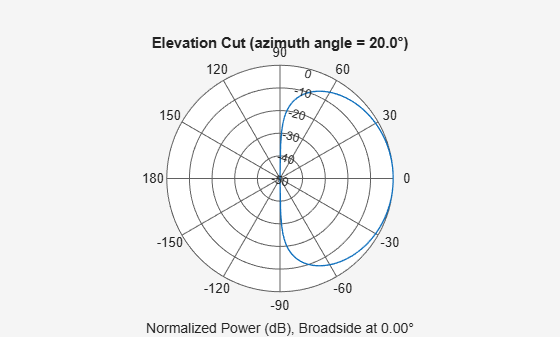Plot an elevation cut of the directivity.

`pattern(antenna,fc,20,-90:90,'CoordinateSystem','polar','Type','directivity')`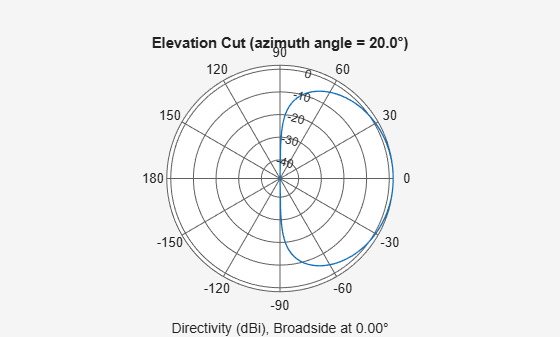Define a custom antenna in u-v space. Then, calculate and plot the response.

Define the radiation pattern (in dB) of an antenna in terms of u and v coordinates within the unit circle.

```u = -1:0.01:1; v = -1:0.01:1; [u_grid,v_grid] = meshgrid(u,v); pat_uv = sqrt(1 - u_grid.^2 - v_grid.^2); pat_uv(hypot(u_grid,v_grid) >= 1) = 0;```

Create an antenna with this radiation pattern. Convert u-v coordinates to azimuth and elevation coordinates.

```[pat_azel,az,el] = uv2azelpat(pat_uv,u,v); array = phased.CustomAntennaElement('AzimuthAngles',az,'ElevationAngles',el, ... 'MagnitudePattern',mag2db(pat_azel),'PhasePattern',45*ones(size(pat_azel)));```

Calculate the response in the direction u = 0.5, v = 0. Assume the antenna operates at 1 GHz. The output of the step method is in linear units.

```dir_uv = [0.5;0]; dir_azel = uv2azel(dir_uv); fc = 1e9; resp = array(fc,dir_azel)```
```resp = 0.6124 + 0.6124i ```

Plot the 3D response in u-v coordinates.

`pattern(array,fc,[-1:.01:1],[-1:.01:1],'CoordinateSystem','uv','Type','powerdb')`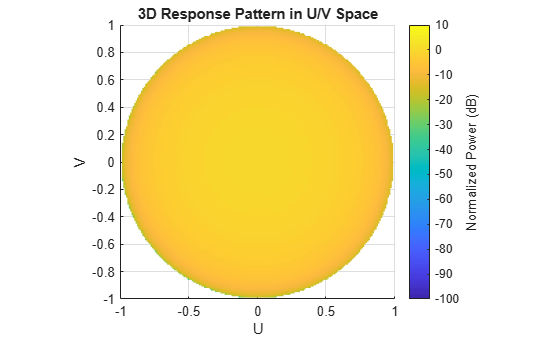Display the antenna response as a line plot in u-v coordinates.

`pattern(array,fc,[-1:.01:1],0,'CoordinateSystem','uv','Type','powerdb')`Model a short dipole antenna oriented along the $x$-axis of the local antenna coordinate system. For this type of antenna, the horizontal and vertical components of the electric field are given by ${E}_{H}=\frac{j\omega \mu IL}{4\pi r}\mathrm{sin}\left(az\right)$ and ${E}_{V}=-\frac{j\omega \mu IL}{4\pi r}\mathrm{sin}\left(el\right)\mathrm{cos}\left(az\right)$.

Specify a normalized radiation pattern of a short dipole antenna terms of azimuth, $az$, and elevation, $el$, coordinates. The vertical and horizontal radiation patterns are normalized to a maximum of unity.

```az = [-180:180]; el = [-90:90]; [az_grid,el_grid] = meshgrid(az,el); horz_pat_azel = ... mag2db(abs(sind(az_grid))); vert_pat_azel = ... mag2db(abs(sind(el_grid).*cosd(az_grid)));```

Set up the antenna. Specify the `SpecifyPolarizationPattern` property to produce polarized radiation. In addition, use the `HorizontalMagnitudePattern` and `VerticalMagnitudePattern` properties to specify the pattern magnitude values. The `HorizontalPhasePattern` and `VerticalPhasePattern` properties take default values of zero.

```antenna = phased.CustomAntennaElement(... 'AzimuthAngles',az,'ElevationAngles',el,... 'SpecifyPolarizationPattern',true,... 'HorizontalMagnitudePattern',horz_pat_azel,... 'VerticalMagnitudePattern',vert_pat_azel);```

Assume the antenna operates at 1 GHz.

`fc = 1e9;`

Display the vertical response pattern.

```pattern(antenna,fc,[-180:180],[-90:90],... 'CoordinateSystem','polar',... 'Type','powerdb',... 'Polarization','V')```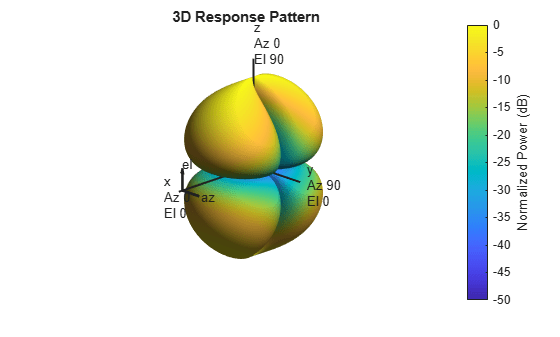Display the horizontal response pattern.

```pattern(antenna,fc,[-180:180],[-90:90],... 'CoordinateSystem','polar',... 'Type','powerdb',... 'Polarization','H')```The combined polarization response, shown below, illustrates the $x$-axis null of the dipole.

```pattern(antenna,fc,[-180:180],[-90:90],... 'CoordinateSystem','polar',... 'Type','powerdb',... 'Polarization','combined')```Define a custom antenna in u-v space. Show how the array response pattern is affected by the choice of the `MatchArrayNormal` property of the `phased.CustomAntennaElement`.

Define the response pattern (in dB) of an antenna as a function of u and v coordinates within the unit circle. The antenna operates at 1 GHz.

```fc = 1e9; c = physconst('LightSpeed'); u = -1:0.01:1; v = -1:0.01:1; [u_grid,v_grid] = meshgrid(u,v); pat_uv = sqrt(1 - u_grid.^2 - v_grid.^2); pat_uv(hypot(u_grid,v_grid) >= 1) = 0;```

Create a custom antenna with this pattern. Convert u-v coordinates to azimuth and elevation coordinates. Set `MatchArrayNormal` to `false`.

```[pat_azel,az,el] = uv2azelpat(pat_uv,u,v); antenna = phased.CustomAntennaElement('AzimuthAngles',az,'ElevationAngles',el, ... 'MagnitudePattern',mag2db(pat_azel),'PhasePattern',45*ones(size(pat_azel)), ... "MatchArrayNormal",false);```

Construct a 3-by-3 URA with this element and display the antenna pattern in 3-D polar coordinates. The element spacing is one-half wavelength. The array normal points along the y-axis.

```lam = c/fc; array = phased.URA('Element',antenna,'Size',[3 3],'ElementSpacing', ... [lam/2 lam/2],'ArrayNormal','y'); pattern(array,fc,-180:180,-90:90,'PropagationSpeed',c, ... 'CoordinateSystem','polar','Type','powerdb','Normalize',true)```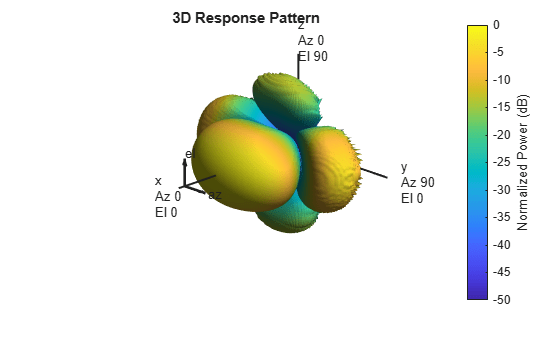The pattern shows the interplay between the element pattern pointing along the x-axis and the array pattern pointing along the y-axis.

Create another custom antenna with the same radiation pattern. Set `MatchArrayNormal` to true. Then create another array with this element.

```antenna2 = phased.CustomAntennaElement('AzimuthAngles',az,'ElevationAngles',el, ... 'MagnitudePattern',mag2db(pat_azel),'PhasePattern',45*ones(size(pat_azel)), ... "MatchArrayNormal",true); array2 = phased.URA('Element',antenna2,'Size',[3 3],'ElementSpacing', ... [lam/2 lam/2],'ArrayNormal','y'); pattern(array2,fc,-180:180,-90:90,'PropagationSpeed',c, ... 'CoordinateSystem','polar','Type','powerdb','Normalize',true)```This pattern shows the aligned element and array patterns pointing along the y-axis.

Construct a user-defined antenna with an omnidirectional response in azimuth and a cosine pattern in elevation. The antenna operates at 1 GHz. Plot the response pattern. Then, find the antenna response at 30°.

```antenna = phased.CustomAntennaElement; antenna.AzimuthAngles = -180:180; antenna.ElevationAngles = -90:90; antenna.MagnitudePattern = mag2db(repmat(cosd(antenna.ElevationAngles)',... 1,numel(antenna.AzimuthAngles)));```

Find the response at 30° elevation for an operating frequency of 1 GHz.

```fc = 1.0e9; resp = antenna(fc,[0;30])```
```resp = 0.8660 ```

Create a custom antenna element object. The radiation pattern has a cosine dependence on elevation angle but is independent of azimuth angle.

```az = -180:90:180; el = -90:45:90; elresp = cosd(el); magpattern = mag2db(repmat(elresp',1,numel(az))); phasepattern = zeros(size(magpattern)); antenna = phased.CustomAntennaElement('AzimuthAngles',az,... 'ElevationAngles',el,'MagnitudePattern',magpattern, ... 'PhasePattern',phasepattern);```

`disp(antenna.MagnitudePattern)`
``` -Inf -Inf -Inf -Inf -Inf -3.0103 -3.0103 -3.0103 -3.0103 -3.0103 0 0 0 0 0 -3.0103 -3.0103 -3.0103 -3.0103 -3.0103 -Inf -Inf -Inf -Inf -Inf ```

Calculate the antenna response at the azimuth-elevation pairs (-30,0) and (-45,0) at 500 MHz.

```ang = [-30 0; -45 0]; resp = antenna(500.0e6,ang); disp(resp)```
``` 0.7071 1.0000 ```

The following code illustrates how nearest-neighbor interpolation is used to find the antenna voltage response in the two directions. The total response is the product of the angular response and the frequency response.

```g = interp2(deg2rad(antenna.AzimuthAngles),... deg2rad(antenna.ElevationAngles),... db2mag(antenna.MagnitudePattern),... deg2rad(ang(1,:))', deg2rad(ang(2,:))','nearest',0); h = interp1(antenna.FrequencyVector,... db2mag(antenna.FrequencyResponse),500e6,'nearest',0); antresp = h.*g;```

Compare the value of `antresp` to the response of the antenna.

`disp(mag2db(antresp))`
``` -3.0103 0 ```

Compute the directivity of a custom antenna element.

Define an antenna pattern for a custom antenna element in azimuth-elevation space. The pattern is omnidirectional in the azimuth direction and has a cosine pattern in the elevation direction. Assume the antenna operates at 1 GHz. Get the response at zero degrees azimuth and from -30 to 30 degrees elevation.

```fc = 1e9; azang = [-180:180]; elang = [-90:90]; magpattern = mag2db(repmat(cosd(elang)',1,numel(azang))); phasepattern = zeros(size(magpattern)); antenna = phased.CustomAntennaElement('AzimuthAngles',azang, ... 'ElevationAngles',elang,'MagnitudePattern',magpattern, ... 'PhasePattern',phasepattern);```

Calculate the directivities as a function of elevation for 0° azimuth angle.

```angs = [0,0,0,0,0,0,0;-30,-20,-10,0,10,20,30]; freq = 1e9; d = directivity(antenna,freq,angs)```
```d = 7×1 0.5115 1.2206 1.6279 1.7609 1.6279 1.2206 0.5115 ```

The directivity is maximum at ${0}^{\circ }$ elevation.

Show that the `CustomAntennaElement` antenna element supports polarization when the `SpecifyPolarizationPattern` property is set to `true`.

```antenna = phased.CustomAntennaElement('SpecifyPolarizationPattern',true); isPolarizationCapable(antenna)```
```ans = logical 1 ```

The returned value `1` shows that this antenna element supports polarization.

Create a custom antenna with a cosine pattern. Show the response at boresight. Then, plot the antenna's field and directivity patterns.

Create the antenna and calculate the response. The user-defined pattern is omnidirectional in the azimuth direction and has a cosine pattern in the elevation direction. Assume the antenna works at 1 GHz.

```fc = 1e9; antenna = phased.CustomAntennaElement; antenna.AzimuthAngles = -180:180; antenna.ElevationAngles = -90:90; antenna.MagnitudePattern = mag2db(repmat(cosd(antenna.ElevationAngles)', ... 1,numel(antenna.AzimuthAngles))); resp = antenna(fc,[0;0])```
```resp = 1 ```

Plot an elevation cut of the magnitude response as a line plot.

```pattern(antenna,fc,0,[-90:90],'CoordinateSystem','rectangular', ... 'Type','efield')```Plot an elevation cut of the directivity as a line plot, showing that the maximum directivity is approximately 2 dB.

```pattern(antenna,fc,0,[-90:90],'CoordinateSystem','rectangular', ... 'Type','directivity')```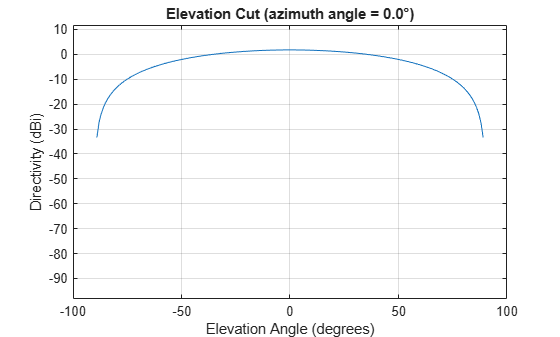Create a custom antenna System object™. The user-defined pattern is omnidirectional in the azimuth direction and has a cosine pattern in the elevation direction. Assume the antenna operates at a frequency of 1 GHz. First show the response at boresight. Display the 3-D pattern for a 60 degree range of azimuth and elevation angles centered at 0 degrees azimuth and 0 degrees elevation in 0.1 degree increments.

```fc = 1e9; azang = -180:180; elang = -90:90; magpattern = mag2db(repmat(cosd(elang)',1,numel(azang))); antenna = phased.CustomAntennaElement('AzimuthAngles',azang, ... 'ElevationAngles',elang,'MagnitudePattern',magpattern); resp = antenna(fc,[0;0])```
```resp = 1 ```

Plot the power pattern for a range of angles.

```pattern(antenna,fc,[-30:0.1:30],[-30:0.1:30],'CoordinateSystem','polar', ... 'Type','power')```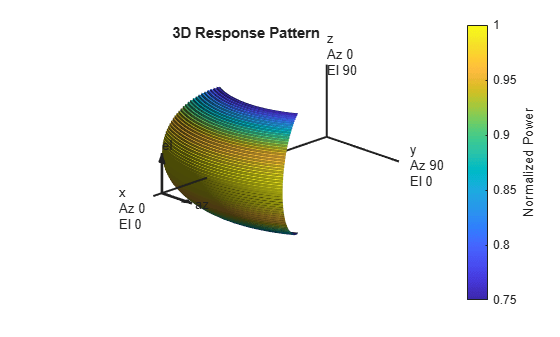Create an antenna with a custom response. The user-defined pattern has a sine pattern in the azimuth direction and a cosine pattern in the elevation direction. Assume the antenna operates at a frequency of 500 MHz. Plot an azimuth cut of the power pattern of the custom antenna element at 0 and 30 degrees elevation. Assume the operating frequency is 500 MHz.

Create the antenna element.

```fc = 500e6; antenna = phased.CustomAntennaElement; antenna.AzimuthAngles = -180:180; antenna.ElevationAngles = -90:90; antenna.MagnitudePattern = mag2db(abs(cosd(antenna.ElevationAngles)'*sind(antenna.AzimuthAngles))); patternAzimuth(antenna,fc,[0 30],'Type','powerdb')```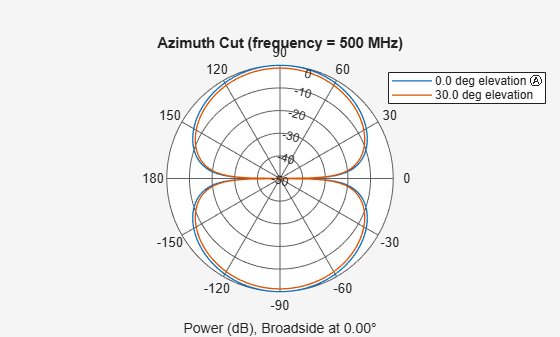Plot a reduced range of azimuth angles using the `Azimuth` parameter.

`patternAzimuth(antenna,fc,[0 30],'Azimuth',[-45:45],'Type','powerdb')`Create an antenna with a custom response. The user-defined pattern has a sine pattern in the azimuth direction and a cosine pattern in the elevation direction. Assume the antenna operates at a frequency of 500 MHz. Plot an elevation cut of the power of the custom antenna element at 0 and 30 degrees elevation. Assume the operating frequency is 500 MHz.

Create the antenna element.

```fc = 500e6; antenna = phased.CustomAntennaElement; antenna.AzimuthAngles = -180:180; antenna.ElevationAngles = -90:90; antenna.MagnitudePattern = mag2db(abs(cosd(antenna.ElevationAngles)'*sind(antenna.AzimuthAngles))); patternElevation(antenna,fc,[0 30],'Type','powerdb')```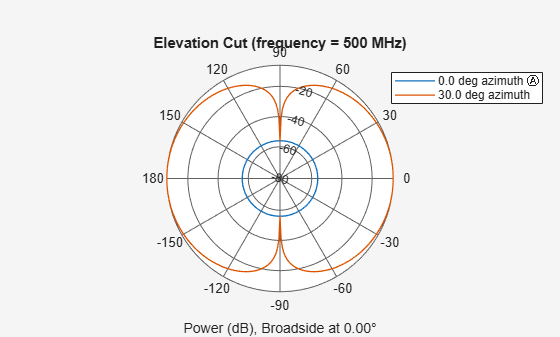Plot a reduced range of elevation angles using the `Azimuth` parameter.

`patternElevation(antenna,fc,[0 30],'Elevation',[-45:45],'Type','powerdb')`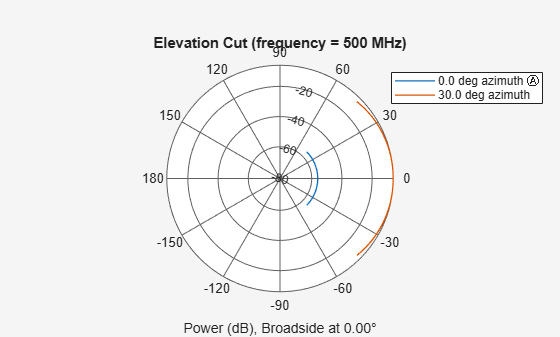## Algorithms

The total response of a custom antenna element is a combination of its frequency response and spatial response. `phased.CustomAntennaElement` calculates both responses using nearest neighbor interpolation, and then multiplies the responses to form the total response.

## Version History

Introduced in R2011a